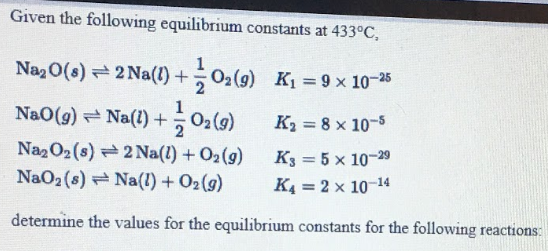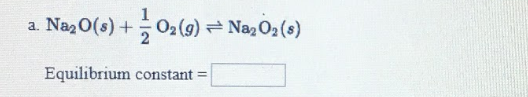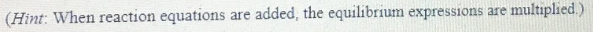# Given the following equilibrium constants at 433 degrees C, determine the values for the equilibrium constants for the following reactions: a) Na2O(s) + 1/2O2(g) ⇌ Na2O2(s) (Hint: When reaction equations are added, the equilibrium expressions are multiplied.)